JEE  >  Doc: Types of Vectors

# Doc: Types of Vectors - Mathematics (Maths) Class 12 - JEE

A vector has direction and magnitude both but scalar has only magnitude.

Magnitude of a vector a is denoted by |a| or a. It is non-negative scalar.

Equality of Vectors

Two vectors a and b are said to be equal written as a = b, if they have (i) same length (ii) the same or parallel support and (iii) the same sense.

Types of Vectors

(i) Zero or Null Vector A vector whose initial and terminal points are coincident is called zero or null vector. It is denoted by 0.

(ii) Unit Vector A vector whose magnitude is unity is called a unit vector which is denoted by nˆ

(iii) Free Vectors If the initial point of a vector is not specified, then it is said to be a free vector.

(iv) Negative of a Vector A vector having the same magnitude as that of a given vector a and the direction opposite to that of a is called the negative of a and it is denoted by —a.

(v) Like and Unlike Vectors Vectors are said to be like when they have the same direction and unlike when they have opposite direction.

(vi) Collinear or Parallel Vectors Vectors having the same or parallel supports are called collinear vectors.

(vii) Coinitial Vectors Vectors having same initial point are called coinitial vectors.

(viii) Coterminous Vectors Vectors having the same terminal point are called coterminous vectors.

(ix) Localized Vectors A vector which is drawn parallel to a given vector through a specified point in space is called localized vector.

(x) Coplanar Vectors A system of vectors is said to be coplanar, if their supports are parallel to the same plane. Otherwise they are called non-coplanar vectors.

(xi) Reciprocal of a Vector A vector having the same direction as that of a given vector but magnitude equal to the reciprocal of the given vector is known as the reciprocal of a.

i.e., if |a| = a, then |a-1| = 1 / a.

## Example 1In the figure given below, identify Collinear, Equal and Coinitial vectors: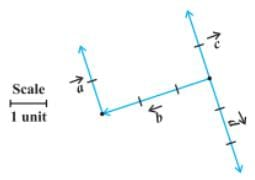Solution: By definition, we know that

• Collinear vectors are two or more vectors parallel to the same line irrespective of their magnitudes and direction. Hence, in the given figure, the following vectors are collinear: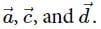• Equal vectors have the same magnitudes and direction regardless of their initial points. Hence, in the given figure, the following vectors are equal: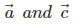• Coinitial vectors are two or more vectors having the same initial point. Hence, in the given figure, the following vectors are coinitial: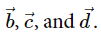More Solved Examples
Question: In the given figure, identify the following vectors

1. Coinitial
2. Equal
3. Collinear but not equal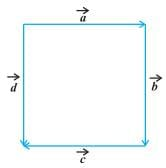Solution:

• Coinitial vectors have the same initial point. In the figure given above, vectors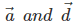are the two vectors which have the same initial point P.
• Equal vectors have same magnitudes and direction. In the figure given above, vectors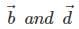are equal vectors.
• Collinear vectors are two or more vectors parallel to the same line. In the figure given above, vectorsare parallel and hence, collinear. Also,  vectorsare parallel and hence, collinear. We know that vectorsare also equal. Hence, vectorsare collinear but not equal.
The document Doc: Types of Vectors | Mathematics (Maths) Class 12 - JEE is a part of the JEE Course Mathematics (Maths) Class 12.
All you need of JEE at this link: JEE

## Mathematics (Maths) Class 12

206 videos|264 docs|139 tests

## FAQs on Doc: Types of Vectors - Mathematics (Maths) Class 12 - JEE

 1. What are the different types of vectors?Ans. In mathematics, there are several types of vectors. Some of the commonly known types include position vectors, displacement vectors, velocity vectors, force vectors, and acceleration vectors.
 2. How are position vectors different from displacement vectors?Ans. Position vectors represent the location of a point in space relative to a reference point, while displacement vectors represent the change in position of an object from its initial position to its final position.
 3. What do velocity vectors represent?Ans. Velocity vectors represent the speed and direction of an object's motion. They indicate how fast an object is moving and in which direction it is moving.
 4. How are force vectors different from acceleration vectors?Ans. Force vectors represent the push or pull acting on an object, causing it to accelerate. On the other hand, acceleration vectors represent the rate of change of velocity of an object with respect to time.
 5. Can vectors have negative magnitudes?Ans. Yes, vectors can have negative magnitudes. The negative sign indicates the opposite direction of the vector. For example, a velocity vector with a negative magnitude indicates motion in the opposite direction compared to the positive magnitude of the same velocity vector.

## Mathematics (Maths) Class 12

206 videos|264 docs|139 testsExplore Courses for JEE examSignup to see your scores go up within 7 days! Learn & Practice with 1000+ FREE Notes, Videos & Tests.
10M+ students study on EduRev
Track your progress, build streaks, highlight & save important lessons and more!
Related Searches

,

,

,

,

,

,

,

,

,

,

,

,

,

,

,

,

,

,

,

,

,

;